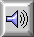SINE WAVE
 Acoustics / Electroacoustics

A sinusoidal wave or function, that is, one moving in SIMPLE HARMONIC MOTION according to the function

A sin (2pft)

where A is the AMPLITUDE of the wave, f its FREQUENCY, and t is time.

According to the FOURIER THEOREM, any periodic WAVEFORM may be analyzed as the sum of a series of sine waves with frequencies in a HARMONIC SERIES, each of which has an amplitude and phase angle given by the Fourier coefficients. Since a sine wave has only a single frequency associated with it, it may be considered the simplest sound.Two CYCLEs of a sine wave showing the amplitude of the pressure variation.Sound Example: Sine wave at 100 Hz.

home